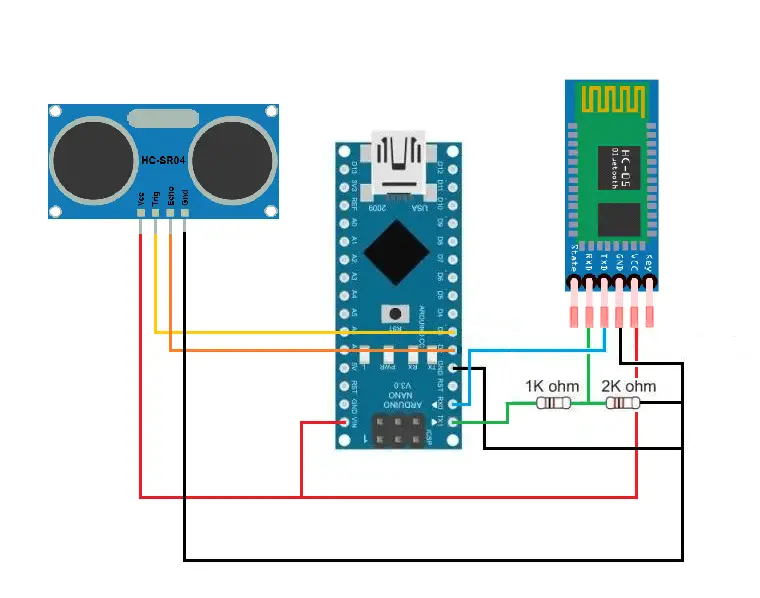# IOT Garbage Monitoring System using Arduino with Bluetooth Application

IOT Garbage Monitoring System using Arduino with Bluetooth Application

Title of the project

IOT Garbage Monitoring System using Arduino with Bluetooth Application

Description

This Garbage Monitoring System will allow user to indicate the amount of trash inside the garbage bin through mobile application.

Schematic DiagramGarbage Monitoring System in Arduino – Schematic Diagram

List of parts and estimated prices (we will update this section)

• Ultrasonic Sensor – \$3.95
• Bluetooth Module (HC-05 or 06) – \$7.99
• Arduino Nano – \$2.05
• Jumper Wires (Female to Female) – \$3.50
• Resistors (1K & 2K ohm) – \$1.0
• Garbage Bin (optional)

How the project works

An Ultrasonic Sensor will measure the depth of the garbage bin, detect the level of the garbage then compile that information to the Arduino, the Arduino will then compute this given information and send it as data to our mobile application

The code below shows how we can use ultrasonic sensor to measure the height of the garbage bin by centimetre (cm).

```const int trigPin = 3; //trigpin of the ultrasonic sensor is connected to pin 3
const int echoPin = 2; //echopin of the ultrasonic sensor is connected to pin 2
long duration, distance, range; //declare long variables to store data

void setup() {
Serial.begin(9600); //initialize serial communication
pinMode(trigPin, OUTPUT); //set trigpin as an OUTPUT
pinMode(echoPin, INPUT); //set echopin as an INPUT
}

void loop() {
digitalWrite(trigPin, LOW); //assure the trigpin is clear
delayMicroseconds(2);
digitalWrite(trigPin, HIGH); //generate ultrasound wave
delayMicroseconds(10);
digitalWrite(trigPin, LOW);
duration = pulseIn(echoPin, HIGH); //read the travel time and store that value in a variable named duration
distance = (duration / 2) / 29.1; //convert duration to centimeters
}

```

To further get a specific result we must convert centimeter to a percentage value

add an integer variable named “Limit” with the height of the garbage bin as its value.

```int Limit = 27; //height limit of your garbage bin in cm
```

We can now convert centimeter into a percentage value by dividing the garbage bin’s height and multiplying it to 100.

`distance = (duration / 2) / 29.1 / Limit * 100; //convert centimeter to a percentage value`

We can then get the range by subtracting the value of percentage to 100.

``` distance = (duration / 2) / 29.1 / Limit * 100; //convert centimeter to a percentage value
range = 100 - distance; //get the range```

Just by checking the value of range we can now send data based on the depth of the garbage bin but first let’s check the value of range if it is between 0% – 100%, this is to ensure the height limit of the garbage bin is correct and not out of range else if it is out of range the Arduino will just send a data “F 100”

```if ((range >= 0) && (range <= 100))
{
}
else
{
Serial.print("F 100");
Serial.println();
delay(500);
}```

Inside the if statement, we can add more if functions to indicate the value of range, if the value range is greater than 70% the Arduino will send “F “ indicating that the garbage bin is Full, if the value range is between 30% to 70% the Arduino will send “M “ indicating that the garbage bin is at the Moderate State and if the value range is less than 30% the Arduino will send “E “ indicating that the garbage bin is Empty while also sending the specific value of range

```if ((range >= 0) && (range <= 100))
{
if (range > 70) //if the range is greater than 70% the Arduino will send “F “
{
Serial.print("F ");
}
if ((range >= 30) && (range <= 70)) // if the range is between 30% to 70% the Arduino will send “M “
{
Serial.print("M ");
}
if (range <= 30) // if the range is less than 30% the Arduino will send “E “
{
Serial.print("E ");
}
Serial.print(range); //sends the specific value of range
Serial.println();
delay(500);
}

```

you can view this data using Serial Monitor.

The Arduino will send this data via Bluetooth then the mobile application will receive this data and will proceed to show the status of the garbage bin to the user, giving a graphical view with a percentage value while also highlighting the garbage bin in color in order to show specific level.

you can watch the video tutorial on this link:

Author:

Hizon Pastorpili
09653873994

Download the mobile application.

Download Source code

How to make the Application from MIT App Inventor

You may visit our facebook page for more information, inquiries and comments.

Hire our team to do the project.